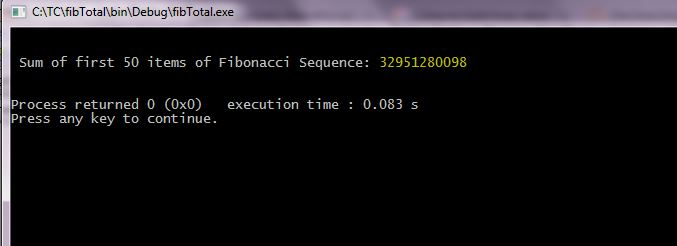# Question & Answer: Write a C program to add the first 50 items of Fibonacci Sequence 1, 1, 2, 3, 5, 8, 13, 21………………50th item…..

Write a C program to add the first 50 items of Fibonacci Sequence 1, 1, 2, 3, 5, 8, 13, 21………………50th item

Don't use plagiarized sources. Get Your Custom Essay on
Question & Answer: Write a C program to add the first 50 items of Fibonacci Sequence 1, 1, 2, 3, 5, 8, 13, 21………………50th item…..
GET AN ESSAY WRITTEN FOR YOU FROM AS LOW AS \$13/PAGE

C Program:

#include <stdio.h>
#include <stdlib.h>

//Main function
int main()
{
//Terms in the series
double f0 = 1, f1 = 1, f2, sum;
int i;

//Calculating sum
sum = f0 + f1;

//Generating and printing from third term
for(i=3; i<=50; i++)
{
//Finding third term
f2 = f0 + f1;

//Updating sum
sum = sum + f2;

//Swapping first and second term with third term
f0 = f1;
f1 = f2;
}

//Calling function and printing result
printf(“nn Sum of first 50 items of Fibonacci Sequence: %.f nn”, sum);

return 0;
}

________________________________________________________________________________________________

Sample Output: